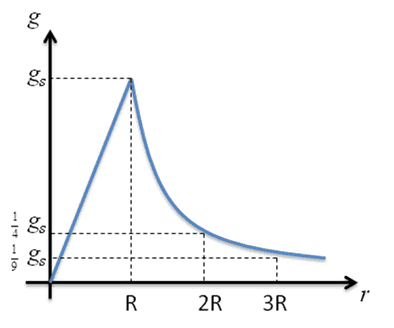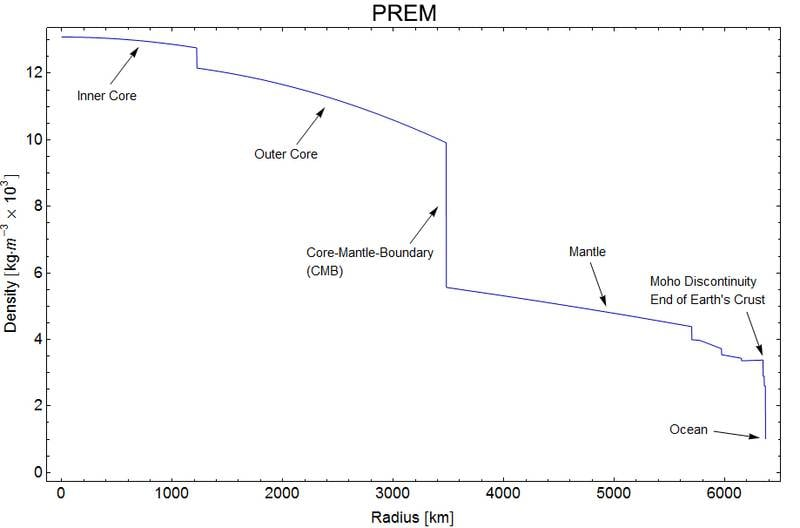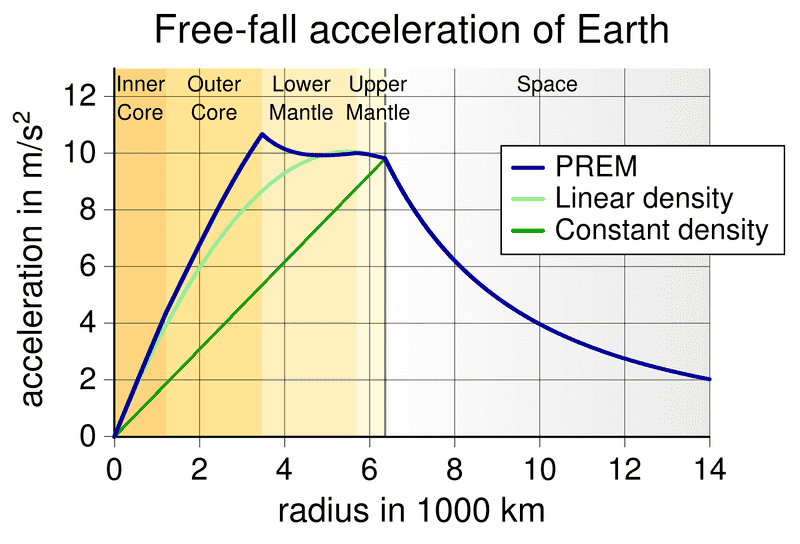# At which point is gravity the strongest?

• B
• Rigel84
Rigel84
I'm embarrassed to admit that I am bit confused with concept of gravity.
Here is practical example: When I stand on surface of the Earth majority, actually all mass is bellow me and gravity pulls me down. Let's imagine now that I am standing on the Earths iron core, majority of mass is above me.
Does gravity works in opposite direction in second case, when I am on the planets core?

Last edited:

Does gravity works in opposite direction in second case, when I am on the planets core?
No, according to Newtons shell theorem (https://en.wikipedia.org/wiki/Shell_theorem) the gravitational field at a point within a uniform spherical mass is only due to the mass at radii below that point. The field produced by the mass at larger radii cancels out.

•Rigel84
For a perfectly uniform density spherical Earth, the gravity is strongest at the surface. But for a realistic Earth, the density is higher deeper underground, so the strongest gravity is somewhere underground. I'm guessing somewhere in the upper mantle, but I'm not sure.

•davenn
Gold Member
I'm embarrassed to admit that I am bit confused with concept of gravity.
Here is practical example: When I stand on surface of the Earth majority, actually all mass is bellow me and gravity pulls me down. Let's imagine now that I am standing on the Earths iron core, majority of mass is above me.
Does gravity works in opposite direction in second case, when I am on the planets core?
The field gets stronger and stronger as you near the surface (inverse square law) and then it decreases proportionally with the distance from the centre. Zero at the centre because less and less of the mass is attracting you..

For a perfectly uniform density spherical Earth, the gravity is strongest at the surface. But for a realistic Earth, the density is higher deeper underground, so the strongest gravity is somewhere underground. I'm guessing somewhere in the upper mantle, but I'm not sure.
It depends on the functional form of the density as a function of distance from the center. If ##\rho(r)## is decays faster than ##1/r^{2}## then the gravity will be stronger underground. If ##\rho(r)## decays slower than ##1/r^{2}## then the gravity will be strongest at the surface. According to this page http://typnet.net/Essays/EarthGrav.htm, it looks like the former is the case and the strongest gravitational field is at just over half the Earth's radius.

•sophiecentaur
Staff Emeritus
I'm embarrassed to admit that I am bit confused with concept of gravity.
Here is practical example: When I stand on surface of the Earth majority, actually all mass is bellow me and gravity pulls me down. Let's imagine now that I am standing on the Earths iron core, majority of mass is above me.
Does gravity works in opposite direction in second case, when I am on the planets core?

What everyone here has posted and has been trying to convey to you is that, IF we assume that the Earth is a sphere (which is not a bad approximation), and if we the mass density is uniform and constant (which it isn't), then gravity inside the Earth will reduce linearly to zero at the very center, i.e.So this is the simplest case. Even if we remove the uniform and constant density, if the mass density can be approximated as being more or less a function of only the radial distance from the center of the earth, then the graph representing g inside the Earth may not be accurate anymore, but the value of gravity is still zero at the very center due to spherical symmetry.

Zz.

#### Attachments

Mark7
Gravity can never be zero at the center of any sphere, only the resultant forces can be zero. Assuming a uniform density, when one is standing on the surface of a sphere the resultant forces are generally pointing towards the center of the sphere because that is where the center of mass is concentrated. At the center of the sphere the gravitational force vectors are from every other possible direction and the gravitational forces balance out, the resultant gravitational force is zero. This is really only true for a infinitely small point within the center of the sphere.

•David Lewis and Sean Nelson
Rigel84
What everyone here has posted and has been trying to convey to you is that, IF we assume that the Earth is a sphere (which is not a bad approximation), and if we the mass density is uniform and constant (which it isn't), then gravity inside the Earth will reduce linearly to zero at the very center, i.e.

View attachment 220006

So this is the simplest case. Even if we remove the uniform and constant density, if the mass density can be approximated as being more or less a function of only the radial distance from the center of the earth, then the graph representing g inside the Earth may not be accurate anymore, but the value of gravity is still zero at the very center due to spherical symmetry.

Zz.

So what causes huge pressure at the Earths core is force cased by attraction of two opposite points? Even if gravity is zero at center or near zero pressure is still high simply because point A is attracted to point B on the other side?
Do I understand this correctly?

Homework Helper
So what causes huge pressure at the Earths core is force cased by attraction of two opposite points? Even if gravity is zero at center or near zero pressure is still high simply because point A is attracted to point B on the other side?
Do I understand this correctly?
One should distinguish between gravitational acceleration, gravitational force, gravitational potential and the pressure due to gravity.

Gravitational acceleration is how rapidly an object would free fall at a particular locationl
Gravitational force is the net force of gravity on an object at a particular location -- gravitational acceleration times the object mass.
Gravitational potential is the potential energy due to that force. It is the integral of the gravitational acceleration over a path to a chosen point of zero potential.
Pressure from gravity is, roughly speaking, the cumulative weight per unit area of all of stuff above a particular point. It is the integral of gravitational acceleration times local density over a path from a point of zero pressure.

willoughby
Yes, there is a difference between what a net force on you would be (and by extension, acceleration) and the pressure. Imagine that you are between two solid iron walls and each wall is exerting a force on you of some arbitrarily high amount. A million tons each, let's say. The net force on you is zero. Your acceleration is zero. But you will be crushed beyond recognition. The same thing would happen in Earth's core. While YOUR weight is zero, the weight of everything around you is not, and it would be exerting an incredible amount of force down on you.

•Sean Nelson and Rigel84
Gold Member
Maybe one day we will reach Mach 30+. Then we can test it!

f todd baker
Uniform density is a quite poor model of the earth. http://askthephysicist.com/images/earthdensity.html Because the core is so much more dense than the mantle and crust, the (calculated) acceleration due to gravity is actually about constant about 1/3 of the way to the center where it increases slightly and then falls fairly linearly to zero at the center.

f todd baker